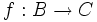# Convention:Function composition

In Groupprops, the notation$f \circ g$ always denotes$g$, followed by$f$. In other words, if$g:A \to B$, and$f:B \to C$ are maps, then$f \circ g$ denotes the composite map from$A$ to$C$.